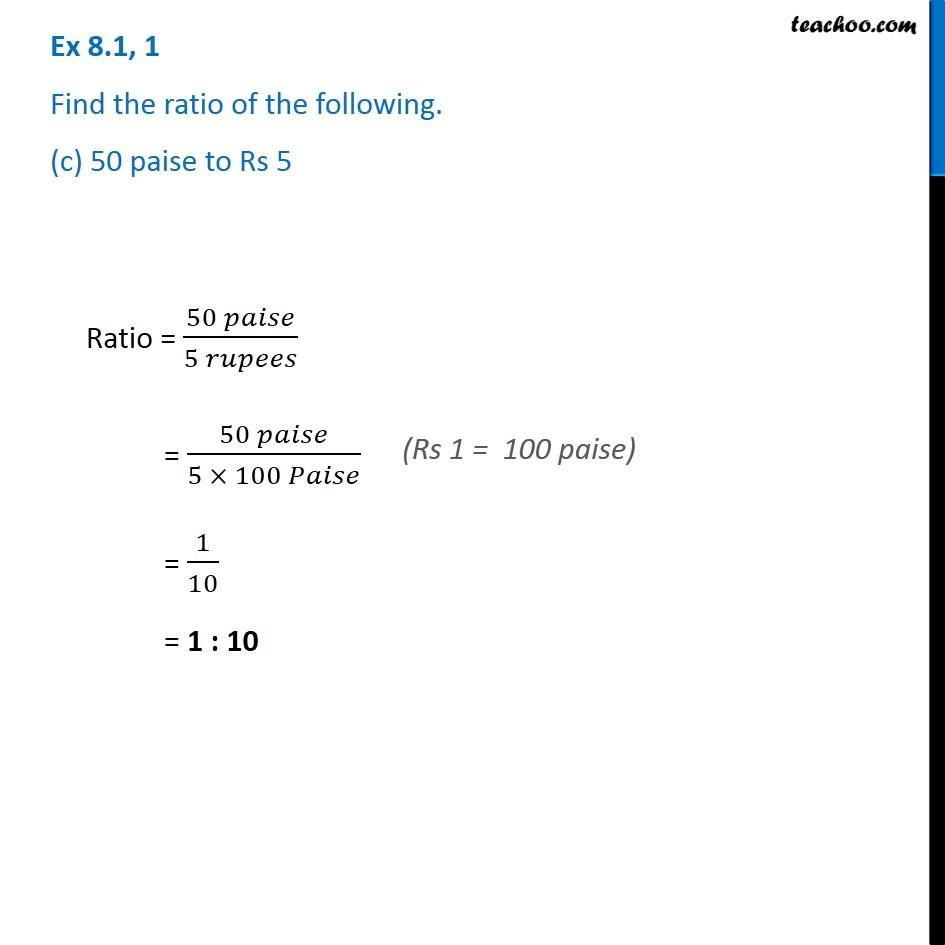Ex 8.1

Chapter 8 Class 8 Comparing Quantities
Serial order wiseGet live Maths 1-on-1 Classs - Class 6 to 12

### Transcript

Ex 8.1, 1 Find the ratio of the following. (c) 50 paise to Rs 5 Ratio = (50 𝑝𝑎𝑖𝑠𝑒)/(5 𝑟𝑢𝑝𝑒𝑒𝑠) = (50 𝑝𝑎𝑖𝑠𝑒)/(5 × 100 𝑃𝑎𝑖𝑠𝑒) = 1/10 = 1 : 10 (Rs 1 = 100 paise)